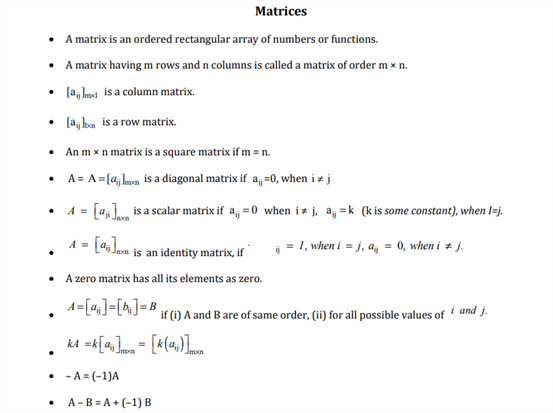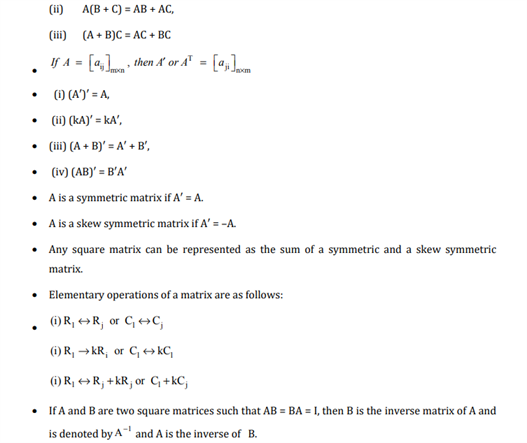# Maths Revision Notes for Class 12 Chapter 3 Matrices.

Chapter 3 revision notes for class 12 maths is available here. We will take a closer look at this chapter to understand the concepts of Matrices better. Some of the major topics introduced in this chapter are the applications and uses of matrices. This particular concept of Matrices is incredibly useful as it has many different uses such as 3D math, cryptography and has many different applications in other branches of science such as genetics, economics, sociology etc. Other major topics taught in this chapter are the definition of matrix, rows of matrix, columns of matrix and elements of matrix as well.

Find the free PDF of class 12 Maths revision notes of chapter 3 Matrices below: